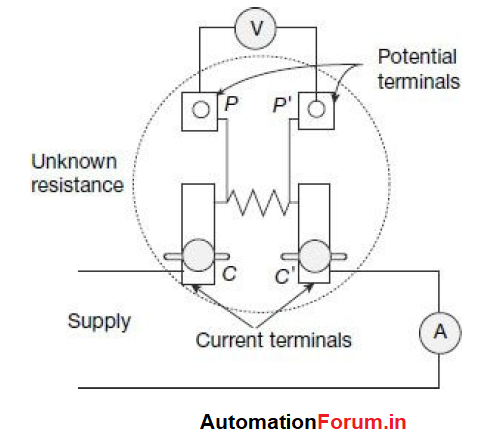# Low resistance measurement techniqueThe methods used for measurement of medium resistances are not suitable for measurement of low resistance. This is due to the fact that resistances of leads and contacts, though small, are appreciable in comparison to the low resistances under measurement.

### Voltmeter–Ammeter Method for Measuring Low Resistance

This method, due to its simplicity, is very commonly used for measurement of low resistances when accuracy of the order of 1% is sufficient. The resistance elements, to be used for such measurements, however, need to of special construction. Low resistances are constructed with four terminals as shown in:One pair of terminals CC’, called the current terminals, is used to lead current to and from the resistor. The voltage drop across the resistance is measured between the other pair of terminals PP’, called the potential terminals. The voltage indicated by the voltmeter is thus simply the voltage drop of the resistor across the potential terminals PP’
and does not include any contact resistance drop that may be present at the current terminals CC’.

Contact drop at the potential terminals PP’ are, however, less itself, since the currents passing through these contacts are extremely small (even zero under ‘null’ balance condition) owing to high resistance involved in the potential circuit.

Value of the unknown resistance RX, in this case, is given by: Voltmeter reading/ ammeter reading

### Kelvin’s Double-Bridge Method for Measuring Low ResistanceKelvin’s double-bridge method is one of the best available methods for measurement of low resistance. It is actually a modification of the Wheatstone bridge in which the errors due to contacts and lead resistances can be eliminated.

Kelvin’s double bridge incorporates the idea of the second set of ratio arms, namely, p and q, and hence the name ‘double bridge. The underbalanced condition, potentials at the nodes a and b must be equal in order that the galvanometer G gives “null” deflection. Since at balance, no current flows through the galvanometer, it can be considered to be open circuited.

### Potentiometer Method for Measuring Low ResistanceThe unknown resistance X is connected in series with a standard known resistance S. Current through the ammeter in the circuit is controlled by a rheostat. A two-pole double throw switch is used. When the switch is in the position 1-1’, the unknown resistance X gets connected to the potentiometer, whereas when the switch is at position 2-2’, the
standard resistance S gets connected to the potentiometer.

Thus, with the switch in position 1-1’, the potentiometer reading is the voltage drop across the unknown resistance, given by Vx= I*X

Scroll to Top